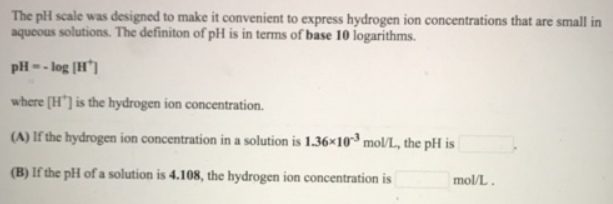# Problem: The pH scale was designed to make it convenient to express hydrogen ion concentrations that are small in aqueous solutions. The definition of pH is in terms of base 10 logarithms. pH = - log [H +] where [H+] is the hydrogen ion concentration. (A) If the hydrogen ion concentration in a solution is 1.36 x 10 -3 mol/L the pH is __________. (B) If the pH of a solution is 4.108, the hydrogen ion concentration is _________ mol/L.

###### FREE Expert Solution
92% (389 ratings)###### Problem Details

The pH scale was designed to make it convenient to express hydrogen ion concentrations that are small in aqueous solutions. The definition of pH is in terms of base 10 logarithms.

pH = - log [H +

where [H+] is the hydrogen ion concentration.

(A) If the hydrogen ion concentration in a solution is 1.36 x 10 -3 mol/L the pH is __________.

(B) If the pH of a solution is 4.108, the hydrogen ion concentration is _________ mol/L.